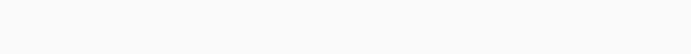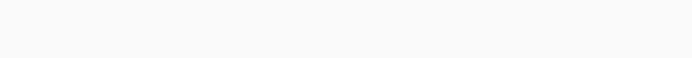#Machine Input-Output Based on SBI PO Mains – Set 29

Hello Aspirants.

Welcome to Online Reasoning Section with explanation in AffairsCloud.com. Here we are creating Best question samples from Machine Input-output, which is common for all the IBPS, SBI , LIC, and other competitive exams. We have included questions that are repeatedly asked in bank exams !!!

I. Study the following information carefully and answer the given questions.
A number arrangement machine arranges two digit numbers into a typical manner. Each step takes gives output taking input from the previous step. The following is an illustration of Input and rearrangement. Using the illustration answer the question given below.

Example:Input:Step I: Multiply the first digit of first number with second digit of fourth Number. Multiply the second digit of first number with first digit fourth number.

Step II: Add the first digit of all numbers in Step I for the first number and second digit of all numbers in Step I for the second number

Step III: Divide second digit by first digit

Step IV: Second number is subtracted from the first number.

1. If the value “5” is subtracted from the final output then what will be the resultant value?
A. -1
B. 1
C. -11
D. 11
E. None of these

2. If in the first step the first digit of every number is added and multiplied by 4 then which will be the resultant value?
A. 56
B. 60
C. 52
D. 64
E. None of these

3. Which of the following combinations represent the first digit of the second value and the second digit of the first value in Step I of the given input?
A. 6, 4
B. 4, 6
C. 8, 2
D. 2, 8
E. 2, 4

4. Which of the following represents the sum of the first digit of the second value and the second digit of the first value in Step II of the given input?
A. 8
B. 7
C. 6
D. 4
E. 9

5. Which of the following represents the difference between the first value and the second value of Step III of the given input?
A. 8
B. 7
C. 9
D. 4
E. 6

I. Study the following information carefully and answer the given questions.
A number arrangement machine arranges two digit numbers into a typical manner. Each step takes gives output taking input from the previous step. The following is an illustration of Input and rearrangement. Using the illustration answer the question given below.

Example:Input:Step I: Multiply the first digit of first number with second digit of fourth Number. Multiply the second digit of first number with first digit fourth number.

Step II: Add the first digit of all numbers in Step I for the first number and second digit of all numbers in Step I for the second number and then multiply by 2.

Step III: Divide second digit by first digit

1. If the value “6” is added to the final output then what will be the resultant value?
A. 12
B. 13
C. 10
D. 11
E. None of these

2. If in the first step the second digit of every number is added and divided by 3 then which will be the resultant value?
A. 5
B. 6
C. 2
D. 4
E. None of these

3. Which of the following combinations represent the first digit of the third value and the second digit of the first value in Step I of the given input?
A. 5, 1
B. 1, 5
C. 8, 6
D. 6, 8
E. 8, 8

4. Which of the following represents the sum of the second digit of the second value and the first digit of the first value in Step II of the given input?
A. 8
B. 7
C. 6
D. 4
E. 9